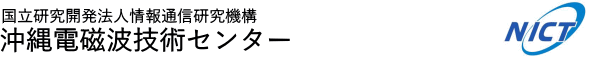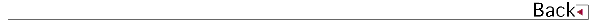\$B!!(B

# 2015\$BG/EY(B (2015.04\$B!A(B2016.03)

## \$B8&5fO@J8(B

• \$BDD(B \$B7K6=(B, \$B;7{(B, Toward Improved Forecasts of Sea-Breeze Horizontal Convective Rolls at Super High Resolutions. Part II: The Impacts of Land Use and Buildings, Monthly Weather Review
• \$BDD(B \$B7K6=(B, \$B;7{(B, Toward Improved Forecast of the Sea-Breeze Horizontal Convective Rolls at Super High Resolution. Part I: Configuration and Verification of the Down-Scaling Simulation System (DS3), Monthly Weather Review

## \$B<}O?O@J8(B

• \$B@nB<(B \$B@?<#(B, \$BB@ED(B \$B90e%G%8%?%kJ|AwGH\$NEAHBCY1d\$rMQ\$\$\$??e>x5\$?dDjpJsDL?.3X2q!!%"%s%F%J!&EAGE8&5f2q(B
• \$B?yC+(B \$BLPIW(B, \$B1+C+(B \$B=c(B, \$B9b@:EYF14|?.9f6!5kAuCV\$rMQ\$\$\$?J,;67?C;GHpJsDL?.3X2q!!1'Ch!&9R9T%(%l%/%H%m%K%/%98&5f2q(B

## \$B0lHL8}F,H/I=(B

• \$BBgLn(B \$BM50l(B, \$BKY9>(B \$B9(><(B, \$B:4F#(B \$B7r<#(B, \$B%"!<%9%1%"1R@1Ek:\1@%l!<%@\$N%S!<%`Fb%(%3!<6/EYIT6Q0l\$N%I%C%W%iJd@5(B(2), \$BF|K\5\$>]3X2q!!=)5(Bg2q(B
• \$BBgLn(B \$BM50l(B, \$BKY9>(B \$B9(><(B, \$B:4F#(B \$B7r<#(B, \$B9b66D*9((B, Evaluation of Doppler Correction Algorithm for EarthCARE Cloud Profiling Radar using Radar Echo Simulation, 37th AMS Conference on radar meteorology
• \$BCf@n(B \$B>!9-(B, \$B2VEZ(B \$B90(B, \$B4d0f(B \$B9(FA(B, \$B9b66(B \$BD*9((B, GPM/DPR Rain Product Validation, AOGS2015
• \$BCf@n(B \$B>!9-(B, \$B2VEZ(B \$B90(B, \$B4d0f(B \$B9(FA(B, \$B9b66(B \$BD*9((B, \$B0f8}(B \$B=SIW(B, Product Validation for GPM/DPR, 7th GPM GV Meeting
• \$BCf@n(B \$B>!9-(B, \$B4d0f(B \$B9(FA(B, \$B@nB<(B \$B@?<#(B, \$B2VEZ(B \$B90(B, \$B0f8}(B \$B=SIW(B, NICT's activity for product validation of GPM/DPR, 2015 NASA PMM Science Team Meeting
• \$BCcLZ(B \$B9E;MO:(B, \$BF#0f(B \$BCR;K(B, \$B?yC+(B \$BLPIW(B, \$B4d0f(B \$B9(FA(B, \$B?9K\(B \$B>e\$K4X\$9\$k8!F\$(B, \$BEE5\$3X2q6e=#;YIt2-Fl;Y=j9V1i2q(B
• \$BBl@n(B \$BE/B@O:(B, \$B?9K\(B \$B>It\$NBPGOCHN.4QB,(B, Asia Oceania Geosciences Society (AOGS)
• \$BBl@n(B \$BE/B@O:(B, \$B>.?K(B \$BE}(B, \$BEOJU(B \$B=S51(B, \$B?9K\(B \$B>B(B \$B85(B, \$B
• \$BBl@n(B \$BE/B@O:(B, \$B>.?K(B \$BE}(B, \$B?9K\(B \$B>B(B \$B85(B, \$Bl\$H%W%i%s%/%H%sJ,I[(B, 2015\$BG/EY6e=#2-FlCO6h9gF1%7%s%]%8%&%`(B
• \$B@nB<(B \$B@?<#(B, \$BB@ED(B \$B905#(B, \$B2VEZ(B \$B90(B, \$B;3K\(B \$B??G7(B, \$B;V2l(B \$B?.BY(B, \$BLZ8M(B \$B9LB@(B, \$B0BED(B \$BE/(B, \$B8eF#(B \$BCi9-(B, \$B1+C+(B \$B=c(B, \$B:#B<(B \$BT"9/(B, \$BF#;^(B \$BH~Jf(B, \$B@PDE(B \$B7rB@O:(B, \$B4d0f(B \$B9(FA(B, \$B?yC+(B \$BLPIW(B, \$BCO>e%G%8%?%kJ|AwGH\$rMQ\$\$\$??e>x5\$?dDj
• \$B@nB<(B \$B@?<#(B, \$BB@ED(B \$B905#(B, \$B2VEZ(B \$B90(B, \$B;3K\(B \$B??G7(B, \$B;V2l(B \$B?.BY(B, \$BLZ8M(B \$B9LB@(B, \$B0BED(B \$BE/(B, \$B8eF#(B \$BCi9-(B, \$B;T@n(B \$BN40l(B, \$B1+C+(B \$B=c(B, \$B:#B<(B \$BT"9/(B, \$BF#;^(B \$BH~Jf(B, \$B@PDE(B \$B7rB@O:(B, \$B4d0f(B \$B9(FA(B, \$B?yC+(B \$BLPIW(B, \$B?e>x5\$4QB,\$rL\E*\$H\$7\$?CO%G%8GHEAHBCY1dB,Dj%7%9%F%`\$N8&5f3+H/(B, \$B5\$>]3X2q(B
• \$B@P0f(B \$B>;7{(B, Baron Philippe, \$B:4F#(B \$BFF(B, \$B2,K\(B \$B9,;0(B, \$B@DLZ(B \$B@?(B, \$BMn9g(B \$B7<(B, \$B?eC+(B \$B9LJ?(B, \$B0B0f(B \$B85><(B, \$B4d0f(B \$B9(FA(B, Sato Masaki, Oki Riko, IWASAKI Toshiki, NISHIZAWA Tomoaki, Technical approaches for future active optical remote sensing, JpGU
• \$B@P0f(B \$B>;7{(B, Baron Philippe, \$B@DLZ(B \$B@?(B, \$BMn9g(B \$B7<(B, \$B?eC+(B \$B9LJ?(B, \$B4d0f(B \$B9(FA(B, \$B0B0f(B \$B85><(B, \$B2C@%(B \$BDgFs(B, \$BB<;3(B \$BJY(B, \$B@u@n(B \$B5AGn(B, \$B:#1|(B \$B5.;V(B, \$BDGL>(B \$BE/CK(B, \$BARED(B \$B8-0l(B, \$B9R6u5!Ek:\%3%R!<%l%s%H:9J,5[<}!&%I%C%W%i!]3X2q(B2015\$BG/EY=U5(Bg2q(B
• \$B@P0f(B \$B>;7{(B, Baron Philippe, \$B:4F#(B \$BFF(B, \$B?eC+(B \$B9LJ?(B, \$B4d0f(B \$B9(FA(B, Toshikazu Itabe, Hirotake Fukuoka, Takayoshi Ishikawa, Kazuhiro Asai, \$B0B0f(B \$B85><(B, \$BMn9g(B \$B7<(B, 2-\$B&L(Bm coherent lidar technology developed at NICT: Past,, Current,, and Future, Application of Lasers for Sensing & Free Space Communication
• \$B@DLZ(B \$B@?(B, \$B4d0f(B \$B9(FA(B, \$BCf@n(B \$B>!9-(B, \$B@P0f(B \$B>;7{(B, \$B?eC+(B \$B9LJ?(B, \$B%3%R!<%l%s%H%I%C%W%i!<%i%\$%@!<\$K\$h\$k1+E)N37BJ,I[\$N1tD>%W%m%U%!%\$%k?dDj(B, \$B%l!<%6!&%l!<%@8&5f2q!!%l!<%6!<%;%s%7%s%0%7%s%]%8%&%`(B
• \$B@DLZ(B \$B@?(B, \$B4d0f(B \$B9(FA(B, \$BCf@n(B \$B>!9-(B, \$B?eC+(B \$B9LJ?(B, \$B%I%C%W%i!<%i%\$%@!<\$K\$h\$k1+E)N37BJ,I[7WB,(B, \$BF|K\5\$>]3X2q(B2015\$BG/EY=U5(Bg2q(B
• \$B@DLZ(B \$B@?(B, \$B4d0f(B \$B9(FA(B, \$BCf@n(B \$B>!9-(B, \$B@P0f(B \$B>;7{(B, \$B?eC+(B \$B9LJ?(B, \$BMn9g(B \$B7<(B, \$B%I%C%W%i!<%i%\$%@!<\$K\$h\$k1+E)N37BJ,I[1tD>%W%m%U%!%\$%k\$N?dDj(B, \$BF|K\5\$>]3X2q(B2015\$BG/EY=)5(Bg2q(B
• \$B@DLZ(B \$B@?(B, \$B4d0f(B \$B9(FA(B, \$BCf@n(B \$B>!9-(B, \$B@P0f(B \$B>;7{(B, \$B?eC+(B \$B9LJ?(B, \$B6a@V30%3%R!<%l%s%H%I%C%W%i!<%i%\$%@!<\$K\$h\$kIw\$H1+\$N7WB,(B, \$BF|K\@V30@~3X2q(B
• \$B?yC+(B \$BLPIW(B, \$B4d0f(B \$B9(FA(B, \$B1+C+(B \$B=c(B, \$B?9K\(B \$B>
• \$B:4F#(B \$B?82p(B, \$B0kED(B \$BAm;R(B, \$B2VEZ(B \$B90(B, \$B4d0f(B \$B9(FA(B, \$BCf@n(B \$B>!9-(B, \$B?eC+(B \$BJ8I'(B, \$B5mHx(B \$BCNM:(B, \$B;09%(B \$B7z@5(B, \$B%U%'!<%:%I%"%l%\$5\$>]%l!<%@!<\$N%G!<%?IJ]3X2q(B2015\$BG/EY=)5(Bg2q(B
• \$B:4F#(B \$B?82p(B, \$B0kED(B \$BAm;R(B, \$B4d0f(B \$B9(FA(B, \$BCf@n(B \$B>!9-(B, \$B2VEZ(B \$B90(B, \$B9b66(B \$BD*9((B, \$B?eC+(B \$BJ8I'(B, \$B5mHx(B \$BCNM:(B, \$B;09%(B \$B7z@5(B, \$B%U%'!<%:%I%"%l%\$5\$>]%l!<%@!<\$K\$h\$k(B30\$BICKh\$N(B3\$B]3X2q(B2015\$BG/EY=U5(Bg2q(B
• \$B4d0f(B \$B9(FA(B, \$B;3ED(B \$B9-9,(B, \$B%i%8%*%>%s%G\$rMQ\$\$\$?(BPAWR\$B\$N%I%C%W%i!Z(B, \$BF|K\5\$>]3X2qJ?@.(B27\$BG/EY2-Fl8&5fH/I=2q(B
• \$B4d0f(B \$B9(FA(B, \$BCf@n(B \$B>!9-(B, \$B2VEZ(B \$B90(B, \$B%U%'!<%:%I%"%l%\$5\$>]%l!<%@\$N%l!<%@H?Z(B, \$BF|K\5\$>]3X2q(B2015\$BG/EY=U5(Bg2q(B
• \$B4d0f(B \$B9(FA(B, \$B@DLZ(B \$B@?(B, \$B%I%C%W%i!<%i%\$%@!<\$K\$h\$j4QB,\$5\$l\$?3\$>eIwCf\$NGH>u9=B\$(B, \$BF|K\5\$>]3X2q(B2015\$BG/EY=)5(Bg2q(B
• \$BCcLZ(B \$B9E;MO:(B, \$BF#0f(B \$BCR;K(B, \$B?yC+(B \$BLPIW(B, \$B4d0f(B \$B9(FA(B, \$B?9K\(B \$B>e\$K4X\$9\$k8!F\$(B, \$BEE5\$3X2q6e=#;YIt2-Fl;Y=j9V1i2q(B
• \$BBl@n(B \$BE/B@O:(B, \$B>.?K(B \$BE}(B, \$BEOJU(B \$B=S51(B, \$B?9K\(B \$B>B(B \$B85(B, \$B
• \$BBl@n(B \$BE/B@O:(B, \$B?9K\(B \$B>It\$NBPGOCHN.4QB,(B, Asia Oceania Geosciences Society (AOGS)
• \$BBl@n(B \$BE/B@O:(B, \$B>.?K(B \$BE}(B, \$B?9K\(B \$B>B(B \$B85(B, \$Bl\$H%W%i%s%/%H%sJ,I[(B, 2015\$BG/EY6e=#2-FlCO6h9gF1%7%s%]%8%&%`(B
• \$B@nB<(B \$B@?<#(B, \$BB@ED(B \$B905#(B, \$B2VEZ(B \$B90(B, \$B;3K\(B \$B??G7(B, \$B;V2l(B \$B?.BY(B, \$BLZ8M(B \$B9LB@(B, \$B0BED(B \$BE/(B, \$B8eF#(B \$BCi9-(B, \$B;T@n(B \$BN40l(B, \$B1+C+(B \$B=c(B, \$B:#B<(B \$BT"9/(B, \$BF#;^(B \$BH~Jf(B, \$B@PDE(B \$B7rB@O:(B, \$B4d0f(B \$B9(FA(B, \$B?yC+(B \$BLPIW(B, \$B?e>x5\$4QB,\$rL\E*\$H\$7\$?CO%G%8GHEAHBCY1dB,Dj%7%9%F%`\$N8&5f3+H/(B, \$B5\$>]3X2q(B
• \$B@nB<(B \$B@?<#(B, \$BB@ED(B \$B905#(B, \$B2VEZ(B \$B90(B, \$B;3K\(B \$B??G7(B, \$B;V2l(B \$B?.BY(B, \$BLZ8M(B \$B9LB@(B, \$B0BED(B \$BE/(B, \$B8eF#(B \$BCi9-(B, \$B1+C+(B \$B=c(B, \$B:#B<(B \$BT"9/(B, \$BF#;^(B \$BH~Jf(B, \$B@PDE(B \$B7rB@O:(B, \$B4d0f(B \$B9(FA(B, \$B?yC+(B \$BLPIW(B, \$BCO>e%G%8%?%kJ|AwGH\$rMQ\$\$\$??e>x5\$?dDj
• \$B?yC+(B \$BLPIW(B, \$B1+C+(B \$B=c(B, \$BL5@~J}<0\$K\$h\$k5?;w;(2;?.9f\$rMQ\$\$\$?9b@:EYF14|?.9f6!5kAuCV(B, \$BEE5\$!&>pJs4X783X2q6e=#;YItBh(B68\$B2sO"9gBg2q(B
• \$B?yC+(B \$BLPIW(B, \$B4d0f(B \$B9(FA(B, \$B1+C+(B \$B=c(B, \$B?9K\(B \$B>
• \$B2H?9(B \$B=SI'(B, \$B>.EDLZ(B \$BMN;R(B, \$B@D;3(B \$BCi;J(B, \$BCf@>(B \$BK.?N(B, \$B2#;3(B \$B2B90(B, \$B:4G<(B \$B9/<#(B, \$B?yC+(B \$BLPIW(B, \$BBgLn(B \$BIR8w(B, \$B0f8}(B \$B@5?M(B, SWARM\$B1R@1<'>l4QB,\$+\$i?dDj\$7\$?2e<'>l\$*\$h\$SHy5\$05JQF0%9%Z%/%H%k\$H\$NHf3S(B, \$BCO5eEE<'5\$!&CO5eOG@17w3X2q!!Am2q5Z\$S9V1i2q(B (SGEPSS)
• T. Iyemori, K. Nakanishi, T. Aoyama, H. Luhr, Y. Odagi, Y. Yokoyama, M. Iguchi, \$B?yC+(B \$BLPIW(B, H. Hashiguchi, M. Utsugi, T. Ono, Y. Sanoo, Time scale of FAC variations estimated by SWARM and a comparison with ground based geomagnetic and micro-barometric observations, AGU\$B!!(BAmerican Geophysical Union Fall Meeting

## 1990\$B!A(B1991\$B!d(B1992\$B!d(B1993\$B!d(B1994\$B!d(B1995\$B!d(B1996\$B!d(B1997\$B!d(B1998\$B!d(B 1999\$B!d(B2000\$B!d(B2001\$B!d(B2002\$B!d(B2003\$B!d(B2004\$B!d(B2005\$B!d(B2006\$B!d(B2007\$B!d(B 2008\$B!d(B2009\$B!d(B2010\$B!d(B2011\$B!d(B2012\$B!d(B2013\$B!d(B2014\$B!d(B2015\$B!d(B2016\$B!d(B2017